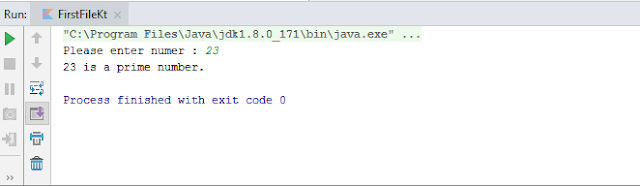# Program to check number is prime or not in kotlin

In this article, we will write a program to check whether the entered number is prime or not in Kotlin Programming.

1. Kotlin Program to check prime number using for loop
import java.lang.Integer.parseInt

fun main(args: Array<String>)
{
var isNumber = true

try
{
val number = parseInt(intInput)
}
catch (e: NumberFormatException)
{
isNumber = false
}

if(isNumber)
{
val num = intInput.toInt()
var flag = false
for (i in 2..num / 2)
{
// condition to check prime number
if (num % i == 0) {
flag = true
break
}
}

if (!flag)
println("\$num is a prime number.")
else
println("\$num is not a prime number.")
}
else
{
println("\$intInput is not a number, Please enter valid input.")
}
}
Output:
21 is not a prime number.Kotlin program to check number is prime or not using for loop - output

Description:
1. In this program, we have taken input from the user using readLine() method.
2. Next validate enter input using parseInt() method, whether enter input is number or not.
2.1. If an entered input is not number, it will just print that "Entered text is not a number, please enter valid input.".
3. Next, inside the for loop, it will check whether the number is divisible by any number in the given range (2..num/2).
4. It is divisible, a flag will set to true and it will break out the loop.
5. if a flag is set to false then the number is prime, else it's not.

2. Kotlin Program to check prime number using a while loop
import java.lang.Integer.parseInt

fun main(args: Array<String>) {
var isNumber = true

try
{
val number = parseInt(intInput)
}
catch (e: NumberFormatException)
{
isNumber = false
}

if(isNumber)
{
val num = intInput.toInt()
var i = 2
var flag = false
while (i <= num / 2) {
// condition for nonprime number
if (num % i == 0) {
flag = true
break
}
++i
}

if (!flag)
{
println("\$num is a prime number.")
}
else
{
println("\$num is not a prime number.")
}
}
else
{
println("\$intInput is not a number, Please enter valid input.")
}
}
Output:Kotlin program to check number is prime or not using while loop - output